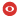Statut Confirmé Série IPN-X Domaines hep-ph Date Jeudi 10 Janvier 2019 Heure 11:00 Institut CPHT Salle Salle de conférences Louis Michel, bât. 6 Nom de l'orateur Hoyer Prenom de l'orateur Paul Addresse email de l'orateur Institution de l'orateur University of Helsinki Titre Bound states and Perturbation theory Résumé Bound states have $O(\alpha^\infty)$ wave functions. Their perturbative expansion is defined by the choice of initial (lowest order) approximation. Initial bound states with valence constituents are appropriate for atoms and motivated by the quark model for QCD. The interactions can be determined by the Hamiltonian in temporal ($A^0 = 0$) gauge, where Gauss law is imposed as a constraint on physical states. The color electric field of a $|q^C \bar q^C>$ meson Fock component is non-vanishing for each quark color $C$, but cancels in the sum over $C$ for color singlet bound states. This allows a homogeneous solution of Gauss law in QCD, with a boundary condition that would be unphysical in QED. Poincare invariance restricts the homogeneous solution up to a universal parameter $\Lambda$, which characterizes the (spatially constant) energy density of the gauge field. The $q\bar q$ Fock component is bound by a linear potential. The potentials for $qqg$, $qqq$, $gg$ and other Fock states are similarly determined. The valence bound states determine the leading contributions to binding energies, since higher Fock states are suppressed by powers of the coupling. The successful phenomenology of heavy quarkonia is reproduced by the $O(\alpha_s^0)$ linear potential and $O(\alpha_s^1)$ gluon exchange. The method applies also to light (relativistic) quarks, revealing linear Regge trajectories with daughters. There are massless $q\bar q$ bound states whose mixing with the vacuum cause a spontaneous breaking of chiral symmetry. Numéro de preprint arXiv Commentaires Fichiers attachés 2019-01-10_slides.pdf (9460165 bytes)Pour obtenir l' affiche de ce séminaire : [ Postscript | PDF ]

 [ Annonces ]    [ Abonnements ]    [ Archive ]    [ Aide ]    [ JavaScript requis ] [ English version ]